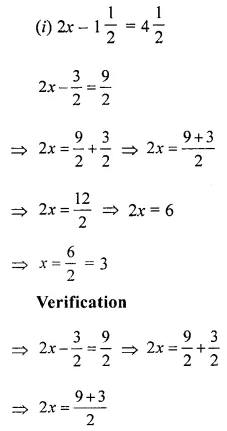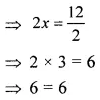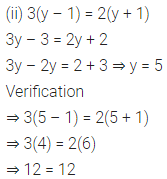# ML Aggarwal Class 6 Solutions for ICSE Maths Chapter 9 Algebra Check Your Progress

## ML Aggarwal Class 6 Solutions for ICSE Maths Chapter 9 Algebra Check Your Progress

Question 1.
Look at the following matchstick pattern of polygons. Complete the table. Also write the general rule that gives the number of matchsticks.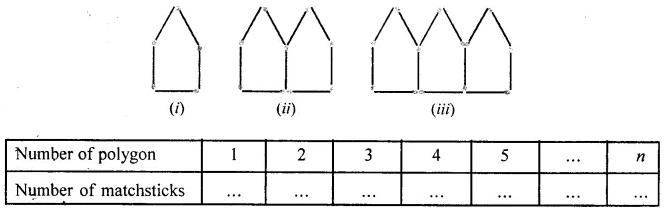Solution: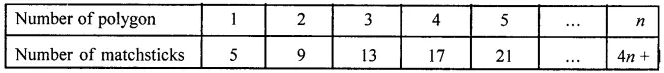Question 2.
Write an algebraic expression for each of the following:
(i) If 1-metre cloth costs ₹ x, then what is the cost of 6-metre cloth?
(ii) If the cost of a notebook is ₹ x and the cost of a book is ₹ y, then what is the cost of 5 notebooks and 2 books?
(iii) The score of Ragni in Mathematics is 23 more than two-third of her score in English. If she scores x marks in English, what is her score in Mathematics?
(iv) If the length of a side of a regular pentagon is x cm, then what is the perimeter of the pentagon?
Solution: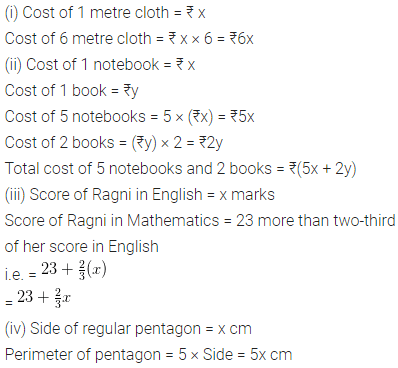Question 3.
When x = 4 and y = 2, find the value of:
(i) x + y
(ii) x – y
(iii) x2 + 2
(iv) x2 – 2xy + y2
Solution: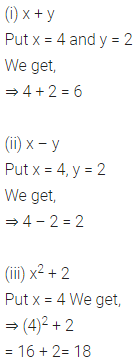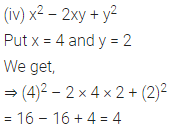Question 4.
When a = 3 and b = -1, find the value of 2a3 – b4 + 3a2b3.
Solution: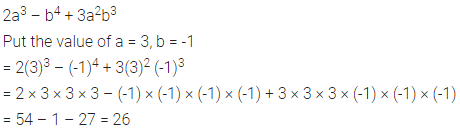Question 5.
When a = 3, b = 0, c = -2, find the values of:
(i) ab + 2bc + 3ca + 4abc
(ii) a3 + b3 + c3 – 3abc
Solution: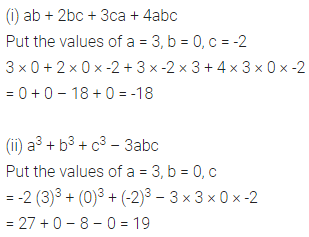Question 6.
Solve the following linear equations:
(i) 2x – $$1 \frac{1}{2}=4 \frac{1}{2}$$
(ii) 3(y – 1) = 2(y + 1)
(ii) n – 3 = 5n + 21
(iv) $$\frac{1}{3}(7 x-1)=\frac{1}{4}$$
Solution: